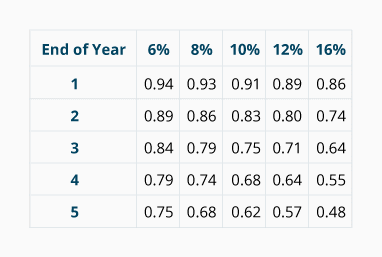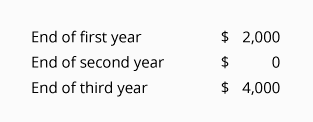# Evaluating Business Investments (Practice Quiz)

For multiple-choice and true/false questions, simply press or click on what you think is the correct answer. For fill-in-the-blank questions, press or click on the blank space provided.

1. Use the following "present value of 1" factors for solving questions that require present value computations.2. 1.

Which of the following models for evaluating capital expenditures considers the time value of money by discounting the future cash flows?

Accounting Rate Of Return
Internal Rate Of Return
Payback
3. 2.

Which of the following models for evaluating capital expenditures does NOT consider the time value of money (does not discount the future cash flows)?

Accounting Rate Of Return
Internal Rate Of Return
Net Present Value
4. 3.

Which of the following models does NOT use cash flows?

Accounting Rate Of Return
Internal Rate Of Return
Payback
5. 4.

A company used the net present value method for evaluating a project. The project requires an immediate cash outlay of \$450,000. The company discounted the cash flows by 16% and determined that the net present value of the project was a negative \$300. From this information it is likely that the project

Had An Internal Rate Of Return That Was Slightly GREATER Than 16%
Had An Internal Rate Of Return That Was Slightly LESS Than 16%
Had A Negative Internal Rate Of Return
6. 5.

A company is planning to invest \$100,000 in order to receive \$50,000 one year later, \$40,000 two years later, and \$60,000 three years later. Using the present value of 1 factors for 16% (see above), the net present value of this investment is

(\$11,000)
\$11,000
\$100,000
\$111,000
7. 6.

A \$100,000 investment will be made on January 1. The cash generated from this investment is expected to be received uniformly during each year. The yearly amounts are: \$50,000 in the first year; \$40,000 in the second year; \$60,000 in the third year. What is the expected payback period?

2.0 Years
2.1 Years
2.17 Years
3 Years
8. 7.

A project's cash flows are discounted by 16% and result is a positive net present value. The positive net present value indicates that the project

Has An Internal Rate Of Return That Is GREATER Than 16%
Has An Internal Rate Of Return That Is LESS Than 16%
9. 8.

Depreciation Expense is a negative cash flow that needs to be discounted.

True
False
10. 9.

While depreciation does not result in a payment of cash, tax depreciation does reduce the cash payments for income taxes.

True
False
11. 10.

Depreciation Expense can be ignored when computing the accounting rate of return.

True
False
12. 11.

If the net present value of a project is \$0, the project should be rejected.

True
False
13. 12.

A project whose future cash flows are discounted by 10% will have a larger net present value than the same cash flows discounted by 8%.

True
False
14. 13.

What amount would you invest today in return for a one-time \$10,000 receipt one year from today, if you want to earn 8%? (Use the factors above.)

\$9,200
\$9,300
\$9,920
15. 14.

How much would you invest today in return for \$20,000 to be received two years from today, if you want to earn 10%? (Use the factors above.)

\$16,000
\$16,600
\$18,260
16. 15.

A potential investment today will provide all of the following cash receipts:If you want to earn a 12% internal rate of return, how much would you invest today to receive these cash amounts? (Use the factors above.)

\$4,260
\$4,620
\$4,980
17. 16.

If you invest \$68,000 today and get repaid \$100,000 at the end of five years, what is the internal rate of return on your investment? (Use the factors above.)

6%
8%
10%
12%
16%
18. 17.

If you invest \$15,900 today and receive \$10,000 at the end of one year plus \$10,000 at the end of four years, what is the internal rate of return on your investment? (Use the factors above.)

6%
8%
10%
12%
16%
19. 18.

If you invest \$15,300 today and receive \$10,000 at the end of one year and \$10,000 at the end of four years, what is the internal rate of return on your investment? (Use the factors above.)

6%
8%
10%
12%
16%
20. 19.

You invest \$10,000 immediately plus an additional \$100,000 at the end of one year. If the time value of money is 10%, what is the present value of your investment? (Use the factors above.)

\$100,000
\$101,000
\$110,000
21. 20.

The state lottery offers a \$500,000 prize consisting of \$100,000 immediately and then four annual payments of \$100,000 each. If the winner prefers to receive an immediate lump sum, the state discounts the future payments by 6%. What will be the immediate lump sum amount before any taxes? (Use the factors above.)

\$346,000
\$400,000
\$446,000
\$470,000

Want more practice questions?